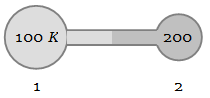In Vander Waal’s equation, a and b represent $\left(\mathrm{P}+\frac{\mathrm{a}}{{\mathrm{V}}^{2}}\right)\left(\mathrm{v}-\mathrm{b}\right)=\mathrm{RT}$

1. Both a and b represent correction in volume

2. Both a and b represent adhesive force between molecules

3. a represents adhesive force between molecules and b correction in volume

4. a represents correction in volume and b represents adhesive force between molecules

Concept Questions :-

Ideal gas
High Yielding Test Series + Question Bank - NEET 2020

Difficulty Level:

At 0°C the density of a fixed mass of a gas divided by pressure is x. At 100°C, the ratio will be:

1. $\mathrm{x}$

2. $\frac{273}{373}\mathrm{x}$

3. $\frac{373}{273}\mathrm{x}$

4. $\frac{100}{273}\mathrm{x}$

Concept Questions :-

Ideal gas
High Yielding Test Series + Question Bank - NEET 2020

Difficulty Level:

At what temperature volume of an ideal gas at 0°C becomes triple :

1. 546°C

2. 182°C

3. 819°C

4. 646°C

Concept Questions :-

Types of velocity
High Yielding Test Series + Question Bank - NEET 2020

Difficulty Level:

If an ideal gas has volume V at 27°C and it is heated at a constant pressure so that its volume becomes 1.5V. Then the value of final temperature will be

1. 600°C

2. 177°C

3. 817°C

4. None of these

Concept Questions :-

Ideal gas
High Yielding Test Series + Question Bank - NEET 2020

Difficulty Level:

A tyre kept outside in sunlight bursts off after sometime because of :

1. Increase in pressure

2. Increases in volume

3. Both (a) and (b)

4. None of these

Concept Questions :-

Specific heat
High Yielding Test Series + Question Bank - NEET 2020

Difficulty Level:

In Boyle's law what remains constant :

1. $\mathrm{PV}$

2. $\mathrm{TV}$

3. $\frac{\mathrm{V}}{\mathrm{T}}$

4. $\frac{\mathrm{P}}{\mathrm{T}}$

Concept Questions :-

Ideal gas
High Yielding Test Series + Question Bank - NEET 2020

Difficulty Level:

Equation of gas in terms of pressure (P), absolute temperature (T) and density (d) is :

1. $\frac{{\mathrm{P}}_{1}}{{\mathrm{T}}_{1}{\mathrm{d}}_{1}}=\frac{{\mathrm{P}}_{2}}{{\mathrm{T}}_{2}{\mathrm{d}}_{2}}$

2. $\frac{{\mathrm{P}}_{1}{\mathrm{T}}_{1}}{{\mathrm{d}}_{1}}=\frac{{\mathrm{P}}_{2}{\mathrm{T}}_{2}}{{\mathrm{d}}_{2}}$

3. $\frac{{\mathrm{P}}_{1}{\mathrm{d}}_{2}}{{\mathrm{T}}_{1}}=\frac{{\mathrm{P}}_{2}{\mathrm{d}}_{1}}{{\mathrm{T}}_{1}}$

4. $\frac{{\mathrm{P}}_{1}{\mathrm{d}}_{1}}{{\mathrm{T}}_{1}}=\frac{{\mathrm{P}}_{2}{\mathrm{d}}_{2}}{{\mathrm{T}}_{2}}$

Concept Questions :-

Ideal gas
High Yielding Test Series + Question Bank - NEET 2020

Difficulty Level:

The figure shows two flasks connected to each other. The volume of the flask 1 is twice that of flask 2. The system is filled with an ideal gas at temperature 100 K and 200 K respectively. If the mass of the gas in flask 1 be m, then what is the mass of the gas in flask 2?1. $\mathrm{m}$

2. $\frac{\mathrm{m}}{2}$

3. $\frac{\mathrm{m}}{4}$

4. $\frac{\mathrm{m}}{8}$

Concept Questions :-

Types of velocity
High Yielding Test Series + Question Bank - NEET 2020

Difficulty Level:

The absolute temperature of a gas is determined by

1. The average momentum of the molecules

2. The velocity of sound in the gas

3. The number of molecules in the gas

4. The mean square velocity of the molecules

Concept Questions :-

Types of velocity
High Yielding Test Series + Question Bank - NEET 2020

Difficulty Level:

At what temperature is the root mean square velocity of gaseous hydrogen molecules is equal to that of oxygen molecules at 47°C

1.  20 K

2.  80 K

3.  – 73 K

4.  3 K

Concept Questions :-

Types of velocity